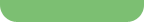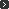공지사항 글보기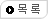작성일2008-03-05 12:31:11 추천/조회수0 / 32767
글쓴이인포컴 E-mailwebmaster@infocomm.co.kr
홈페이지http://www.infocomm.co.kr
제목LDPC - Another Key Step Toward Shannon

New low-density parity check (LDPC) error correction techniques push wireless and networking channel performance closer to the Shannon Limit. Here's how.

 By Tony Summers, Comtech AHA Corporation

For communication designers, especially those in the networking and wireless field, the Shannon limit can be seen as the Holy Grail. And, since being first defined in the late 1940s, designers have developed and implemented error correction coding techniques to push channel performance closer and closer to the Shannon limit.

For the past few years, turbo coding was discussed and touted as the key FEC technique for improving channel performance. Now, a new technique, called low-density parity check (LDPC), is emerging and could replace turbo coding as the FEC of choice by taking designers even closer to the Shannon Limit.

In this tutorial, we'll take a look at the key elements that make LDPC error coding come to life. We'll then show how LDPC can be used in a communication system design.

Comparing FEC Techniques
Forward-error correction (FEC) is valued in communication links because it allows for virtually error free communications over a noisy channel. FEC improves system capacity by permitting high data rates within the communications link while providing improved transmission power efficiency. This relationship is referred to as the channel capacity for additive white gaussian noise (AWGN) channels and is measured by the famous formula developed by Shannon:

C = W log2(1 + P/N)

where:
C is capacity (bits per second)
W is the bandwidth (Hertz)
P is transmitter power (Watts)
N is the noise (Watts)

The challenge of developing new coding solutions is to provide performance gains closer to the channel capacity while not limiting throughput or adding latency. Claude Shannon showed that it is possible to optimize the energy and time transmitting data across a communications channel if you have the right coding scheme.

Before Shannon's work, it was thought that to reduce communications errors, either transmission power must be increased or the message data must be sent repeatedly. By the early 1990s, the best practical coding solutions resulted in performance 3 to 5 dB away from the channel capacity, or the Shannon Limit.

In 1993, a significant breakthrough occurred when two French electrical engineers, Claude Berrou and Alain Glavieux discovered turbo codes. Their claim was that their turbo coding scheme would result in a 3 dB performance improvement over the best competing FEC coding solutions in existence. The claims were so unbelievable that many coding experts refused to even read the IEEE paper presenting the codes at the Conference on Communications in Geneva, Switzerland. Later, other researchers began to replicate the results and realized the significance of this discovery.

Turbo codes come in two flavors that are very different in structure: turbo convolutional codes (TCCs) and turbo product codes (TPCs). TCCs use parallel convolutional encoders and an interleaver to generate the parity bits. These are the Turbo Codes discovered by Berrou and Glavieux.

In contrast to TCCs, TPCs use extended hamming codes and parity codes as constituent codes to build 2D and 3D block structures. This results in a coding scheme that is far less complex to decode than the TCCs and is scalable to easily support the full range of data rate requirements up to gigabits per second. The benefit of this is smaller, less expensive decoders for cost sensitive applications.

After the turbo codes started to gain attention in the comm sector, designers turned their FEC research efforts toward the area of soft decision iterative decoders and toward searching for lower complexity codes. These efforts led to a rediscovery of LDPC codes, first proposed by Robert Gallager1 in his 1960 doctoral dissertation. Robert Tanner2, in 1981 generalized LDPC codes and developed a graphical method of representing these codes, now called Tanner graphs or bipartite graphs. Other than Tanner's important work, little was done with LDPC codes until David MacKay3, Michael Luby4, and others resurrected them in the mid-1990s. The LDPC codes have resulted in FEC solutions that perform even closer to the Shannon Limit. An LDPC code is based on an H matrix containing a low count of ones. Encoding is done by using equations derived from the H matrix to generate the parity check bits. Decoding is accomplished using "soft-inputs" with these equations to generate new estimates of the sent values. This process is repeated in an iterative manner resulting in a very powerful decoder. LDPC codes can be subject to error floors, as is common with TCCs. To address the error floor, an outer code, such as Bose-Bhaudhuri-Hocquenghem (BCH), can be added to LDPC technology. The BCH outer code has the effect of lowering the error floor. Digital Video Broadcast (DVB) has chosen this method of FEC for its new DVB-S2 standard.

With the flexibility of LDPCs, codes can be constructed to exactly match a particular block size or code rate, though practical implementations may impose certain constraints on block sizes and/or obtainable code rates. After the block size and code rate are established, an H matrix is constructed that is n columns wide by (n-k) rows high and contains a sparse number of ones.

A properly designed H matrix should have a large minimum distance (dmin). This is a result of having a low number of ones in the H matrix and as such the number of columns of H required to sum to zero tends to be high even for randomly constructed codes. The minimum distance for the following example code is only four, as can be seen by looking at columns 0, 1, 3, and 4 of the H matrix.

Encoding Example A simple LDPC encoder can be demonstrated using a simple (16,9) code with the following H matrix. Parameters for this example are:

k message bits = 9
n-k parity bits = 7
Code Rate = k/n = 9/16In the code example above, columns n0 through n8 represent the message part of the encoded block, while columns n9 through n15 represent the (n-k) parity bits and form a submatrix that is lower triangular in nature further simplifying the encoding process. For example, encoding parity bit n9 only requires knowledge of n0, n1, and n2. Mod-2 logic equations can be written directly from the rows of this H matrix to form the LDPC encoder as follows:

Write a parity equation for the first row to encode n9.

n0 + n1 + n2 + n9 = 0
n9 = n0 + n1 + n2

Continue with the remaining rows to obtain the full 7 equations.

n9 = n0 + n1 + n2
n10 = n3 + n4 + n5
n11 = n6 + n7 + n8
n12 = n0 + n3 + n6
n13 = n1 + n4 + n7
n14 = n2 + n5 + n8
n15 = n12 + n13 + n14

To complete an LDPC encoder, designers need to convert each mod-2 logic equation above to a circuit comprising a three input exclusive or XOR gate and then register the outputs. For a systematic code, the order of the bits sent into the channel should be maintained as n0 ... n15. Using this method of encoding eliminates the need to explicitly determine the generator matrix and subsequently using the generator matrix equations to encode the data.

The LDPC Decoder
The LDPC decoder receives blocks of data, including corrupted bits due to noise, with five or six bits resolution of confidence information for each 0 or 1 bit received. Decoding a block uses an iterative process that consists of solving the (n-k) parity check equations of the H matrix. Solving the equations in this case means updating the confidence that the bits in the equations are ones or zeroes using belief propagation or simplified approximations of belief propagation. This is repeated for many iterations, often 30 to 60, to fully decode each block of received data.

The decoder can stop once a valid codeword is found (satisfying all parity check equations) or when the allotted time has been spent without finding a codeword. Large block sizes and extra iterations improve the performance of the codes, but both add to latency, data rate, and memory size issues.

Comparing LDPC codes with TPCs and other standard coding options shows gains of more than 0.5 dB from a low code rate TPC, and up to 2 dB from other coding solutions. TPC 16K block size codes perform close to the LDPC codes at code rates approaching 0.9. The modulation used in this comparison is binary phase-shift keying (BPSK) and the channel is AWGN (Figure 1).Figure 1: Comparing LDPC with TPC and other coding schemes.

Market Applications
Researchers are investigating the use of advanced error correction coding for digital audio and video broadcasting, as well as for increasing data rates in enhanced versions of wireless LAN (WLAN) wi-Fi networks. LDPC codes are proving to give excellent coding gains over a wide range of code rates and block sizes.

The major benefits from using LDPCs is a performance gain measured in dB that can be used in a variety of ways such as lower transmit power, higher data throughput, longer transmit distances, or increased reliability of the communication link. When transmit power is limited, the extra coding gain of LDPC codes can make the difference between reliable communications and no communication.

Figure 2 shows a simplistic wireless system that implements LDPC FEC and demonstrates its operation. In this figure, a message originates at the computer terminal connected to the transmitter and is separated into k-bit message blocks prior to LDPC encoding. The encoding process adds the n-k parity bits to the end of each message block and as shown using the same 9/16 code and associated H matrix described in the previous section. The modulator transforms and groups the LDPC encoded bits into symbols that are optimized for transmission through the wireless channel.Figure 2: Diagram showing the use of LDPC in a WiFi design.

In Figure 2, received data is demodulated and input to the LDPC decoder which iteratively processes each n-bit block, correcting any bit errors until decoding convergence is reached, or the system has used up the allocated time for processing that particular block. The (n-k) parity bits are then removed and the corrected message block is output to the computer terminal.

Modem Performance
Figures 3 and 4 are screen captures from an oscilloscope taken during lab testing of a new modem design implementing LDPC error correction.

Figure 3 shows a clean constellation where the transmitter is looped back into the receiver with a piece of coax cable. The 16 constellation points of the 16-level quadrature amplitude modulation (16-QAM) constellation are very apparent in this image.Figure 3: Diagram showing a clean 16-QAM channel.

Figure 4 is the constellation with a noisy channel. All of the noise shown in this picture is 100% corrected by the LDPC decoding using a commercially available LDPC encoder and decoder implementation.Figure 4: Diagram showing a noisy 16-QAM channel.

Wrap Up
LDPC codes are a class of linear block codes that are proving to be the best performing forward error correction currently available. Markets such as broadband wireless and mobile networks operate in noisy environments and need powerful error correction in order to improve reliability and throughput data rates. Through LDPC, these systems can operate more reliably, efficiently, and at higher data rates.

References

1. Robert Gallager, "Low-density parity-check codes," IRE Trans. Information Theory, pp. 21-28, Jan. 1962.
2. Robert M. Tanner, "A recursive approach to low complexity codes," IEEE Trans. Inf. Theory, pp. 533-547, Sept. 1981.
3. David MacKay, et al. "Comparison of constructions of irregular Gallager codes," IEEE Trans. Comm. October 1999.
4. Michael Luby et al. "Improved low-density parity-check codes using irregular graphs," IEEE Trans. Inf. Theory, Feb. 2001.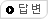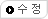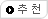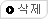번호제목글쓴이등록일추천조회